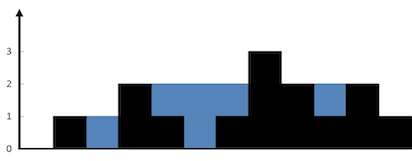# Trapping Rain Water

Given n non-negative integers representing an elevation map where the width of each bar is 1, compute how much water it is able to trap after raining.image was borrowed from leetcode

The above elevation map is represented by array [0,1,0,2,1,0,1,3,2,1,2,1]. In this case, 6 units of rain water (blue section) are being trapped. Thanks Marcos for contributing this image!

Example:

Input:

``` [0,1,0,2,1,0,1,3,2,1,2,1]
```

Output:

` 6`

This problem was taken from Leetcode

## Solution

The brute force approach: for each element we go to the right and find the maximum height of the bar, then we go to the left and do the same.

For any element the maximum amount of the water that could be trapped will be the minimum of left height and right height, minus the height of the bar.

So for the array [0,1,0,2,1,0,1,3,2,1,2,1] we go all the way to the right and calculate the max right value, starting from first element ‘0’ max right will be 0. ‘1’ – max right is ‘1’ and so on.
We repeat the same from last element ‘1’ to the first one.

Then the trapped water for the first column will be:  min(maxRight, maxLeft) – theArrayElement[n]

 the array 0 1 0 2 1 0 1 3 2 1 2 1 max right 0 1 1 2 2 2 2 3 3 3 3 3 max left 3 3 3 3 3 3 3 3 2 2 2 1 collected water 0 0 1 0 1 2 1 0 0 1 0 0The complexity will be O(n2)

```/**
* @param {number[]} height
* @return {number}
*/
var trap = function(height) {
if(height.length < 2)
return 0;

let findMaxLeft = function(idx, height) {
let max = 0;
for(let i =idx;i >= 0; i --) {
max = Math.max(max, height[i]);
}
return max;
}

let findMaxRight = function(idx, height) {
let max = 0;
for(let i = idx;i < height.length; i ++) {
max = Math.max(max, height[i]);
}
return max;
}

let collectedWater = 0;
for(let i = 0;i < height.length; i ++) {

const maxLeft = findMaxLeft(i, height);
const maxRight = findMaxRight(i, height);

let min = Math.min(maxLeft, maxRight);
collectedWater += (min - height[i]);
}

return collectedWater;
};```

The better solution: find all max left and max right with one loop, then do a second loop for each element in the array, and calculate trapped water.

```/**
* @param {number[]} height
* @return {number}
*/
var trap = function(height) {
let maxLeftArray = [], maxRightArray = [];
let maxLeft = 0, maxRight = 0;
const ln = height.length;
let trappedWater = 0;

for(let i = 0;i < height.length; i ++) {
maxLeftArray[i] = Math.max(height[i], maxLeft);
maxLeft = maxLeftArray[i];

maxRightArray[ln - i - 1] = Math.max(height[ln - i - 1], maxRight);
maxRight = maxRightArray[ln - i - 1];
}

for(let i = 0;i < height.length; i ++) {
trappedWater += Math.min(maxLeftArray[i], maxRightArray[i]) - height[i];
}
return trappedWater;

};```
what we just did:

– With one loop find the max left and right bar on each side.
– for any element the maximum amount of the water that could be trapped will be the minimum of left height and right height, minus the height of the bar.